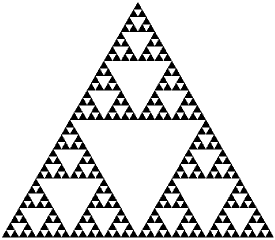#The Sierpinski Gasket

Math Lair Home > Topics > The Sierpinski GasketThe Sierpinski gasket is a type of fractal. There are two versions of this fractal, a square one and a triangular one. The method of creating them is identical: Start with a square or an equilateral triangle. For the equilateral triangle, remove the triangle formed by the midpoints of the triangle, and repeat the process with the remaining three smaller triangles. For the square, remove the central 1/3 square, and repeat the process for the remaining eight squares.

Looking at Pascal's Triangle, if you were to shade all even numbers white and all odd numbers black (see my Pascal's Triangle Java applet to do this online), and zoomed out on it, it would look just like a Sierpinski gasket (unless, of course, if you zoomed back in on it, when it wouldn't show more detail, unlike a Sierpinski gasket, which is a fractal and so would show more detail).

What is the area of the Sierpinski gasket? Well, before the middle triangle is removed, say that it has an area of one unit. Removing the middle triangle reduces its area by a factor of ¼, so there is ¾ units left. Each iteration reduces the area by a factor of ¼, so as the number of iterations approaches infinity, the approaches ¾ × ¾ × ¾ × ¾ ... , which approaches zero. Therefore the area of the Sierpinski gasket is zero. Yet, it still contains an uncountably infinite number of points.